hufang360 的勋章

等级： 0 (0 Points)

100 Points Until Level 1

其他标识

1.正版玩家

• Points
0

活动优胜勋章

1.四周年特别勋章

• Points
0

活动参与勋章

1.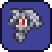社区元老

• Points
0
2.四周年限定勋章

• Points
0
3.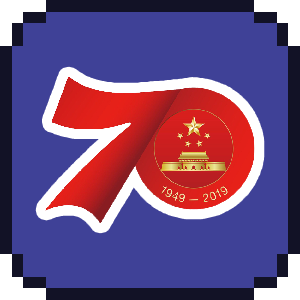新中国成立70周年纪念勋章

• Points
0
4.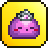旅程的终点

• Points
0
5.五周年限定勋章

• Points
0
6.六周年限定勋章

• Points
0

成就勋章

1.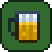来一杯

• Points
0
2.成为爱豆

• Points
0
3.杰出资讯官

• Points
0
4.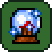杰出技能家

• Points
0
5.杰出宝藏家

• Points
0
6.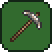杰出程序猿

• Points
0
7.杰出翻译官

• Points
0
8.杰出视觉师

• Points
0
9.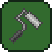杰出设计师

• Points
0
10.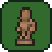杰出创作家

• Points
0
11.• Points
0